## Physics of EEG/MEG

 We consider a head to be  a volume conductor with a nonuniform anisotropic conductivity tensor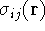, and a magnetic permeability of free space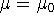. In general, electromagnetic field in the media is described by the system of Maxwell equations: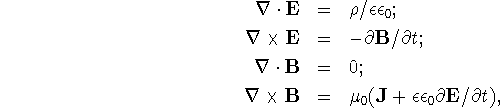where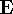and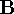are electric and magnetic fields, J is the current density andis the permitivity of the media. Another important equation is the continuity equation: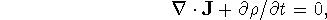where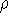is an electric charge density. In neuromagnetism, frequencies are usually below 100Hz and electric and magnetic field time derivatives are typically much smaller than ohmic currents, so we can neglect the displacement current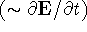and the non-potentiality of thefield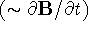. Thus, below we will be using a quasi-static approximation of Maxwell's equations: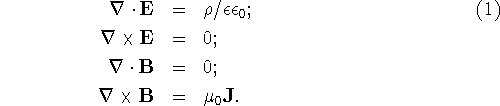and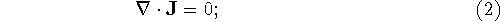(this last equation simply states that the sum of all the currents entering the volume is zero, or Kirkoff's second law). It is convenient to split the total current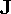into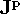- primary current, or current from the sources, and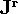, secondary or return current. The primary current(sources) creates electric field which causes secondary current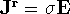. Then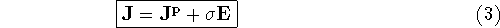Substituting this equation into continuity equation (2), we get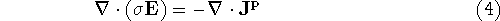Since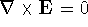, the electric field can be represented as a gradient of some potential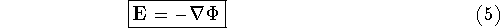Substituting this expression into Eq.(4) we get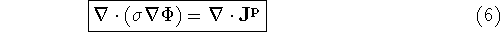which is a Poisson's equation for an electric potential. The second Maxwell's equation, together with the continuity equation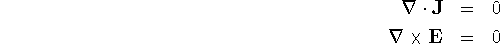provides boundary conditions on the boundary interface between two media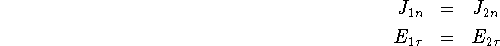Since we consider a boundary between a conducting media and an insulator (in the air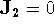), the boundary conditions will read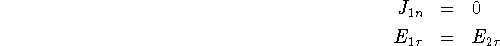Assuming no current sources in the surface layer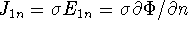. This gives us the boundary condition for the Poisson equation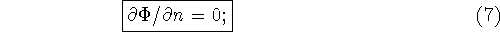on the outside surface (scalp). There is no current outside of the media, but there still exists the tangential electric field which decays with distance. Since the surface shape is non-trivial, the solution of Eq. (6) with boundary conditions Eq. (7) can only be found numerically. Since the divergence of a magnetic field is always zero (no magnetic monopoles exists),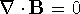, the magnetic field can be represented as a curl of some vector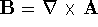, where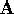is called a vector potential. Substitution of this expression in the last Maxwell's equation leads to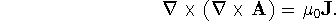Using vector calculus we get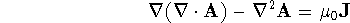Vector potentialis defined up to a gauge. Choosing the ``Coulomb gauge'' we set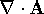=0 and simplify the last equation to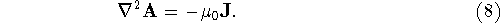This is again a Poisson's equation, but now with a different set of boundary conditions. Since magnetic permeability of the media is the same as of the air, there is no boundary conditions exists for the magnetic field. The only physical condition will be that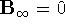, no magnetic field at infinity. In this case the Poisson's equation can be solved analytically and the solution for the vector potential is well known and given by the following integral: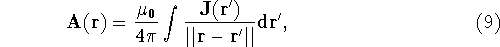where integration is over the entire volume space (currents are non-zero only inside the head!). After taking the curl we get the expression for the magnetic field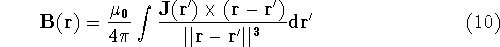Substituting current from Eq.(3,5) into the above formula we get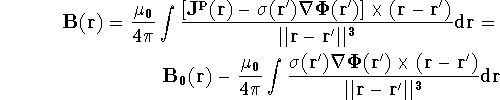where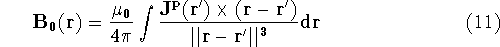Applying differentiation by parts and assuming zero conductivity outside the surface, we can rewrite the above integral as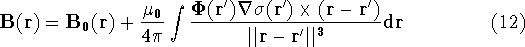It is clear from the last expression, that if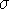were constant, then the magnetic field would only be due to the primary source currents. Ifcan be considered piece-wise constant within certain areas, then the above integral will be non-zero only on the boundary between those areas and can easily be reduced to a sum of the surface integrals over those surfaces. In our case, though,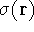is anisotropic and varies continuously in space, so we have to compute the second integral explicitly. The primary current densitycan be approximated by a current dipole which is a unidirectional line element of current pumped from a ``sink'' to a ``source''. The convenient mathematical representation for the the current densitycan be given through the Dirac delta function (point source)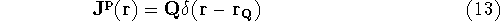where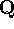is a current dipole. It can also be interpreted as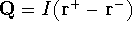- dipole current I times the distance between the ``source'' and the ``sink'. Then the Poisson equation (6) will become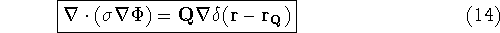Finally, we can summarize the algorithm for the solution of EEG/MEG forward problems: EEG forward problem Set up current sources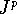; Solve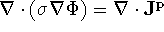with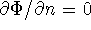boundary conditions; Find potentials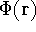on the scalp. MEG forward problem Repeat first two steps from the above; Compute conduction current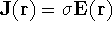Compute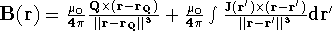; Find components of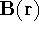field at the desired positions.

Zhukov Leonid
Tue Aug 31 13:36:30 MDT 1999
 Revised: March , 2005